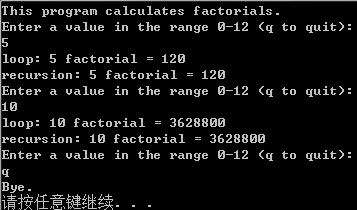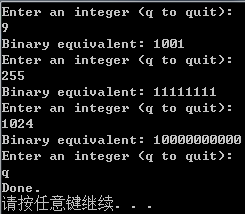﻿ C语言—函数

## C语言—函数

```//Visual Studio中加上这句才可以使用scanf()
//否则只能使用scanf_s()
#define _CRT_SECURE_NO_WARNINGS
#include <stdio.h>
#include <stdbool.h>

#define NAME "GIGATHINK, INC."
#define PLACE "Megapolis, CA 94904"
#define WIDTH 40

void starbar(void); /* ANSI C风格函数原型 */
//void starbar(); /* 对于不识别ANSI C风格的编译器这样声明 */
//一些老的编译器甚至连void都识别不了

//argc: 参数个数 argv[]: 参数数组
int main(int argc, char *argv[])
{
// 也可以在函数里面声明
//void starbar(void);

starbar();
printf("%s\n", NAME);
printf("%s\n", PLACE);
starbar(); /* 调用函数 */

system("pause");
return 0;
}

void starbar(void) /* 定义函数 */
{
int count;
for (count = 1; count <= WIDTH; count++)
putchar('*');
putchar('\n');
}```

```//Visual Studio中加上这句才可以使用scanf()
//否则只能使用scanf_s()
#define _CRT_SECURE_NO_WARNINGS
#include <stdio.h>
#include <stdbool.h>

#define NAME "GIGATHINK, INC."
#define PLACE "Megapolis, CA 94904"
#define WIDTH 40
#define SPACE ' '

// 推荐的声明方式
void show_n_char(char ch, int num);
// 推荐的声明方式，根据个人喜好声明函数时也可以不写变量名
//void show_n_char(char, int);

// 也可以不声明参数（已废弃）
//void show_n_char();

// ANSI C之前的声明形式(已废弃)
//void show_n_char(ch, num)
//char ch;
//int num;

//argc: 参数个数 argv[]: 参数数组
int main(int argc, char *argv[])
{
int spaces;
show_n_char('*', WIDTH);
putchar('\n');
show_n_char(SPACE, 12);
printf("%s\n", NAME);
spaces = (WIDTH - strlen(ADDRESS)) / 2;
show_n_char(SPACE, spaces);
show_n_char(SPACE, (WIDTH - strlen(PLACE)) / 2);
printf("%s\n", PLACE);
show_n_char('*', WIDTH);
putchar('\n');

system("pause");
return 0;
}

void show_n_char(char ch, int num)
{
int count;
for (count = 1; count <= num; count++)
putchar(ch);
}```

```//Visual Studio中加上这句才可以使用scanf()
//否则只能使用scanf_s()
#define _CRT_SECURE_NO_WARNINGS
#include <stdio.h>
#include <stdbool.h>

int imin(int, int);

// ANSI C允许使用部分原型(实用于函数参数不固定的情况)。
// 如： int printf(const char *, ...);

//argc: 参数个数 argv[]: 参数数组
int main(int argc, char *argv[])
{
int evil1, evil2;
printf("Enter a pair of integers (q to quit):\n");
while (scanf("%d %d", &evil1, &evil2) == 2)
{
printf("The lesser of %d and %d is %d.\n",
evil1, evil2, imin(evil1, evil2));
printf("Enter a pair of integers (q to quit):\n");
}
printf("Bye.\n");

system("pause");
return 0;
}

// 如果把函数定义放在main()之前，则可以省略函数声明。
int imin(int n, int m)
{
return (n < m) ? n : m;
}```

```//Visual Studio中加上这句才可以使用scanf()
//否则只能使用scanf_s()
#define _CRT_SECURE_NO_WARNINGS
#include <stdio.h>
#include <stdbool.h>

void up_and_down(int);

//argc: 参数个数 argv[]: 参数数组
int main(int argc, char *argv[])
{
up_and_down(1);
system("pause");
return 0;
}

void up_and_down(int n)
{
printf("Level %d: n location %p\n", n, &n);
if (n < 4)
up_and_down(n + 1);
printf("LEVEL %d: n location %p\n", n, &n);
}```

```//Visual Studio中加上这句才可以使用scanf()
//否则只能使用scanf_s()
#define _CRT_SECURE_NO_WARNINGS
#include <stdio.h>
#include <stdbool.h>

long fact(int n);
long rfact(int n);

//argc: 参数个数 argv[]: 参数数组
int main(int argc, char *argv[])
{
int num;
printf("This program calculates factorials.\n");
printf("Enter a value in the range 0-12 (q to quit):\n");
while (scanf("%d", &num) == 1)
{
if (num < 0)
else if (num > 12)
printf("Keep input under 13.\n");
else
{
printf("loop: %d factorial = %ld\n",
num, fact(num));
printf("recursion: %d factorial = %ld\n",
num, rfact(num));
}
printf("Enter a value in the range 0-12 (q to quit):\n");
}
printf("Bye.\n");

system("pause");
return 0;
}

// 使用循环计算
long fact(int n)
{
long ans;
for (ans = 1; n > 1; n--)
ans *= n;
return ans;
}

// 使用递归计算
long rfact(int n)
{
long ans;
if (n > 0)
ans = n * rfact(n - 1);
else
ans = 1;
return ans;
}``````//Visual Studio中加上这句才可以使用scanf()
//否则只能使用scanf_s()
#define _CRT_SECURE_NO_WARNINGS
#include <stdio.h>
#include <stdbool.h>

void to_binary(unsigned long n);

//argc: 参数个数 argv[]: 参数数组
int main(int argc, char *argv[])
{
unsigned long number;
printf("Enter an integer (q to quit):\n");
while (scanf("%lu", &number) == 1)
{
printf("Binary equivalent: ");
to_binary(number);
putchar('\n');
printf("Enter an integer (q to quit):\n");
}
printf("Done.\n");

system("pause");
return 0;
}

// 使用递归将十进制数用二进制表示
void to_binary(unsigned long n)
{
int r;
r = n % 2;
if (n >= 2)
to_binary(n / 2);
putchar(r == 0 ? '0' : '1');
}```